Mobile QR Code1. (School of Semiconductor and Chemical Engineering, Semiconductor Physics Research Center, Chonbuk National University, Jeonju 54896, Korea.)
2. (R&DDivision, Sigetronics, Inc., Jeollabuk-do 55314, Korea)

$AlGaN$/$GaN$ HEMT, MOS diode, gateleakage mechanisms, Poole-Frenkel emission, Schottky emission mechanism

## I. INTRODUCTION

The AlGaN/GaN HEMTs are extremely promising devices for high power and high frequency device applications due to their properties of high breakdown voltage and high carrier velocity. The high-density and high-mobility two-dimensional electron gas (2DEG) generated at the AlGaN/GaN interface results in the realization of the power switching transistors having extremely low on-state resistance applicable to next generation power conversion systems [1-3]. In switching applications, the reverse gate leakage current will lead to high off-state power consumption and the forward leakage current limits the range of gate voltage swing, hence deteriorating the power handling capability. The gate leakage current is a key factor to assure good power added efficiency and linearity in high power amplifiers and noise figure in low noise amplifiers. However, the high gate leakage currents associated with the strong polarization electric field due to Al rich barriers layers, higher density of surface states limits the performance and reliability of AlGaN/GaN HEMTs . The GaN HEMT (MOSHEMT) using a dielectric film as a gate insulator effectively reduces the high gate leakage current and improves the device performance [5,6]. The gate current is one of the most important parameters for HEMTs/MOSMEMTs. Hence, it is of prime necessary to fully analyze and properly engineer the reverse leakage current in GaN-based HEMTs/MOSHEMTs so as to improve their performance in the high-power device applications. The reverse leakage current is usually dominated by mechanisms such as Poole-Frenkel emission, Schottky emission, direct tunneling, Fowler-Norheim tunneling, and trap-assisted tunneling. Poole-Frenkel emission refers to electric field-enhanced thermal emission of electrons from a trap state into a continuum of electronic states rather than direct thermionic emission from the metal [7,8]. While in Schottky emission, the electron absorbs thermal energy and then emitted over the potential barrier at the interface . In direct tunneling, the electrons tunnel all the way through the barrier and is more dominant in junctions having thinner oxide layers/barriers while the Fowler-Nordheim tunneling occurs in junctions having thicker oxide layers through the triangular barriers. Generally, the tunneling current remains unchanged with variation in temperature. It is reported that the Fowler-Nordheim tunneling possesses a temperature dependence and that the reverse leakage current by the tunneling could also increase with temperature as the ejected electrons follows the Fermi-Dirac distribution [9,10]. Trap-assisted tunneling is a current transfer mechanism in which electrons are assisted by defects/traps in the oxide, to propagate through the insulating layer. In contrast to single step tunneling process like Fowler-Nordheim tunneling or direct tunneling, the traps in oxide layer assist the electrons to tunnel from one electrode side to the other through a two-step process. The electrons are first captured from first electrode side into the traps and subsequently emitted to the other electrode side.

Until now, several attempts have been made to investigate the current transport mechanisms dominating the gate current in conventional AlGaN/GaN HEMTs [11-14]. For instance, Turuvekere $et$ $al$.  investigated the possible gate leakage mechanisms in AlGaN/GaN and AlInN/GaN HEMTS and that the thermionic emission and Poole-Frenkel emission mechanisms are observed in AlGaN/GaN HEMT, with an additional Fowler-Nordheim tunneling component existing in AlInN/GaN HEMT. Further, they observed trap-assisted tunneling current component existing in both the set of devices. Xia $et$ $al$.  studied the reverse leakage current characteristics of Ni Schottky contacts to GaN grown on Si using HEMTs in the temperature range of 273–428 K. They found that the reverse leakage current is dominated by Frenkel-Poole emission when the reverse electric field is < 1.4 MV/cm and by Fowler-Nordheim tunneling mechanism for electric field > 1.6 MV/cm. Yan $et$ $al$.  determined the field-dependent reverse gate leakage current characteristics of AlGaN/GaN HEMTs including the polarization effect within the AlGaN barrier into calculation of the near-surface electric field underneath the Schottky metal. They found that around zero bias, the reverse polarization-field-induced Frenkel-Poole emission current is balanced by a forward defect-assisted tunneling current, both of which follow the same temperature dependent characteristics.

Even though, there are numerous reports available on the conventional Schottky-gate AlGaN/GaN HEMT, the reports on the gate leakage current mechanism in AlGaN/GaN HEMT with gate oxide is quite scarce. In particular, the atomic layer deposited (ALD) Al$_{2}$O$_{3}$ was proven to be an excellent gate dielectric for GaN MOS-HEMTs exhibiting low leakage current, high breakdown voltage, strong accumulation and high effective 2D electron mobility under both low and high transverse fields . Swain $et$ $al$.  has made an attempt to develop an analytical model for gate leakage current during forward bias in an AlGaN/GaN MOSHEMT. They reported that at high temperature ($\textit{T}$ > 388 K), the trap-assisted tunneling (TAT) mechanism dominates the gate leakage current at low electric field for bias from 0 to 2 V, while Poole-Frenkel emission component is dominant during medium and high electric field. During low temperature ($\textit{T}$ < 388 K), TAT alone is dominant and consistent in the whole range of electric field. Liu $et$ $al$.  investigated the mechanisms of the temperature-dependent forward gate current transport in the atomic-layer-deposited (ALD) Al$_{2}$O$_{3}$/AlGaN/GaN metal-insulator-semiconductor high electron mobility transistor (MISHEMT). They found that Fowler-Nordheim tunneling dominates the forward current transport at low temperature ($\textit{T}$ < 0 $^{\circ}$C) and high electrical field and TAT mechanism was dominant at medium electrical field and high temperature ($\textit{T}$ > 0 $^{\circ}$C) as against the domination of thermionic field emission mechanism in conventional Schottky-gate AlGaN/GaN HEMT. Reddy $et$ $al$.  demonstrated dual-surface modification of GaN/AlGaN/GaN high-electron mobility transistors (HEMTs) using tetramethylammonium hydroxide (TMAH) and piranha solutions prior to gate metallization and investigated the gate leakage mechanisms considering Poole-Frenkel and Schottky emission mechanisms.

In this work, we investigate the gate current transport mechanisms of the 25 nm thick ALD Al$_{2}$O$_{3}$ gate oxide AlGaN/GaN MOSHEMTs at different measurement temperatures in the range of 300–400 K. The depletion depth profile and the interface state density have been investigated from the capacitance-voltage characteristics. It will be shown that the Poole-Frenkel emission and Schottky emission mechanisms dominates the reverse leakage current transport regardless of the temperature, in the lower and higher bias regions, respectively. The current transport mechanism dominating the forward bias current have been analyzed.

## II. GROWTH AND DEVICE FABRICATION

Fig. 1. Schematic diagram and optical image of AlGaN/GaN MOS-HEMT fabricated using ALD Al$_{2}$O$_{3}$ gate oxide.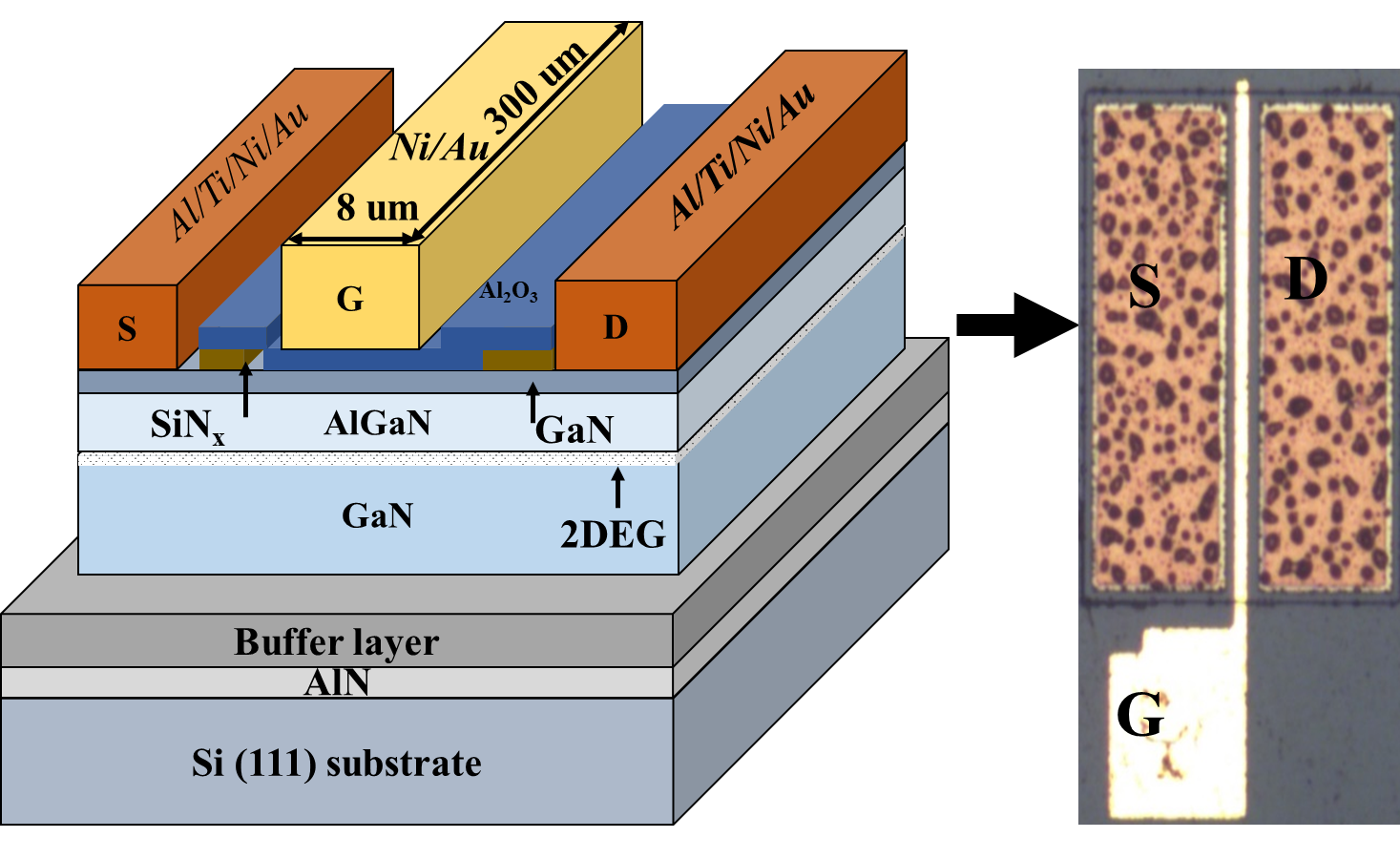Fig.1(a) shows the schematic diagram, and (b) optical images of AlGaN/GaN MOSHEMT with Al$_{2}$O$_{3}$ gate oxide. The AlGaN/GaN heterostructure used in this work was grown by metal-organic chemical vapor deposition on Si (111) substrate. The epitaxial structure consists of a 2 nm thick cap layer, a 25 nm thick AlGaN barrier layer, 300 nm thick undoped GaN, a 20 μm thick buffer layer, and AlN spacer layer. The Al mole fraction of the barrier AlGaN was 22 % for the structures used in this study. Hall measurements at room temperature provided a carrier concentration of 8.6 ${\times}$ 10$^{12}$ cm$^{-3}$ and a carrier mobility of 1638 cm$^{2}$/V${\cdot}$s. The AlGaN/GaN wafers were initially cleaned with organic solvents. The sample surface was treated in a 30 %-HF solution for 5 min for the removal of native oxide. In this study, we employed the “ohmic first” process to prevent damage to the Al$_{2}$O$_{3}$ film during ohmic annealing. Ti/Al/Ni/Au (30/70/30/70 nm) source and drain electrodes were then deposited followed by rapid thermal annealing (RTA) at a temperature of 850 $^{\circ}$C for 2 minutes using N$_{2 }$ambient for alloying. After the contacts were formed, 150 nm thick SiN was deposited using plasma enhanced chemical vapor deposition (PECVD) as a surface protection layer. Then, the mesa isolation was performed in an inductively coupled plasma (ICP) chamber using Cl$_{2}$-based plasma at Radio frequency power/bias power of 200/50 W at 20 sccm flow rate with GXR 601 positive photoresist as mask. The SiN layer was then removed with a buffered HF. A 25 nm thick Al$_{2}$O$_{3}$ gate oxide was then deposited with ALD using trimethylaluminum and water vapor as precursors. Finally, an Au/Ni (50/100 nm) gate electrode was deposited on the Al$_{2}$O$_{3}$ layer. Later, the source-drain contacts have been opened with negative photoresist as mask through etching oxide layer on source-drain region using ICP etcher based on oxide layer etching conditions. In this work, the gate leakage current is measured between source and gate under the gate area of 8 ${\times}$ 300 μm$^2$, with the source-gate distance of 9 μm. The current-voltage ($\textit{I}$–$\textit{V}$) and capacitance-voltage ($\textit{C}$–$\textit{V}$) characteristics of Au/Ni/Al$_{2}$O$_{3}$/AlGaN/GaN MOS diode have been measured using precision semiconductor parameter analyzer (Agilent 4155C), and a precision LCR meter (Agilent 4284A), respectively.

## III. RESULTS AND DISCUSSION

The energy band diagrams of Au/Ni/Al$_{2}$O$_{3}$/AlGaN /GaN MOS diode are presented in Fig.2. The parameters that are required in drawing energy band diagrams include work function, band gap, and electron affinity values of ${\Phi}$$_{Au} = 5.1 eV, {\Phi}$$_{Ni}$ = 5.2 eV, ${\Phi}$$_{AlGaN} = 4.3 eV, {\Phi}$$_{GaN}$$_{\mathrm{ =}} 4.02 eV, \textit{E}$$_{g,Al2O3 }$= 7.0 eV, $\textit{E}$$_{g,AlGaN }= 3.89 eV, \textit{E}$$_{g,GaN }$= 3.4 eV, ${\chi}$$_{\mathrm{AlGaN }}= 3.41 eV, and {\chi}$$_{\mathrm{GaN}}$ =4.02 eV [20-23], respectively. The energy band diagram of this MOS structure consists of Au/Ni metal stacks for gate electrode, Al$_{2}$O$_{3}$ ALD gate oxide, and AlGaN/GaN semiconductor whose operating conditions depend on the applied voltage. Therefore, energy band diagrams have been determined under different bias conditions. As can be seen in Fig.2(a), the energy band diagram of Au/Ni/Al$_{2}$O$_{3}$/AlGaN/GaN MOS diode under gate bias is 0 V (flat-band conditions). When applied bias voltage is 0 V, no charge is present in oxide-semiconductor interface. Also, the Fermi level of Au/Ni gate metal is same as that of the Fermi level of AlGaN/GaN, indicating that the device is in equilibrium condition. Fig.2(b) shows the energy band diagram when the applied gate voltage is negative ($\textit{V}$$_{\mathrm{g }}< 0 V). When the negative voltage is applied, Fermi level of Au/Ni gate metals will move up and its location is above the conduction band of AlGaN while Fermi level of AlGaN/GaN remains constant. Due to this, there is no current flow on AlGaN/GaN side. Therefore, the charge bends the band upwards on the AlGaN/GaN-Al_{2}O_{3} interface. When voltage is applied to the Au/Ni gate metals, negative charge appears on the Au/Ni-Al_{2}O_{3} junction, and excess holes are induced at the AlGaN/GaN-Al_{2}O_{3} interface. In this case, holes accumulate near the semiconductor-oxide interface and can be considered as accumulation conditions. Fig.2(c) shows the band diagram for the positive voltage (\textit{V} > 0). In this case, the Fermi level of Au/Ni gate metal, which has been above the valence band, has been moved down to a location below the valence band. The positive charge appears near the Au/Ni-Al_{2}O_{3} junction causing the electrons to travel towards the gate creating a negative charge on the AlGaN/GaN-Al_{2}O_{3} interface, and the charge bends the band downwards on the AlGaN/GaN-Al_{2}O_{3} interface. The electrons will recombine with the holes that are present near the oxide leading to creation of depletion region. Surface voltage develops in depletion region and as an effect of this, the energy band bends in the depletion region. Fig. 2. Energy band diagrams of Au/Ni/Al_{2}O3/AlGaN/GaN MOS diode under different bias conditions of (a) zero bias, (b) negative bias, (c) positive bias.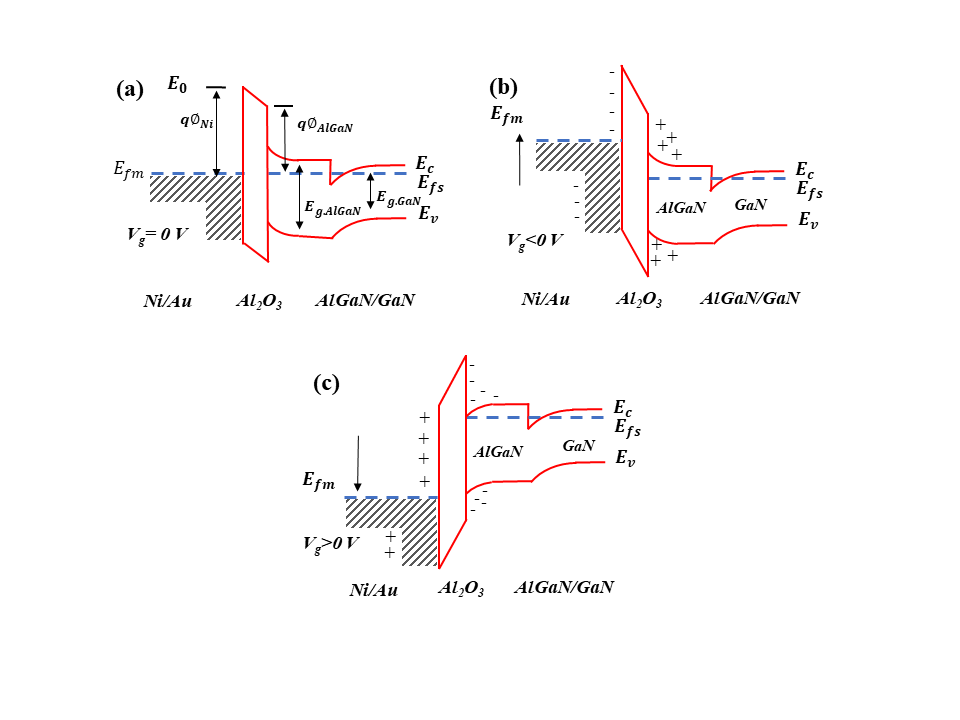Fig. 3. Typical forward and reverse bias I-V characteristics of Au/Ni/Al_{2}O_{3}/AlGaN/GaN MOS diode measured in the temperature range of 300–400 K.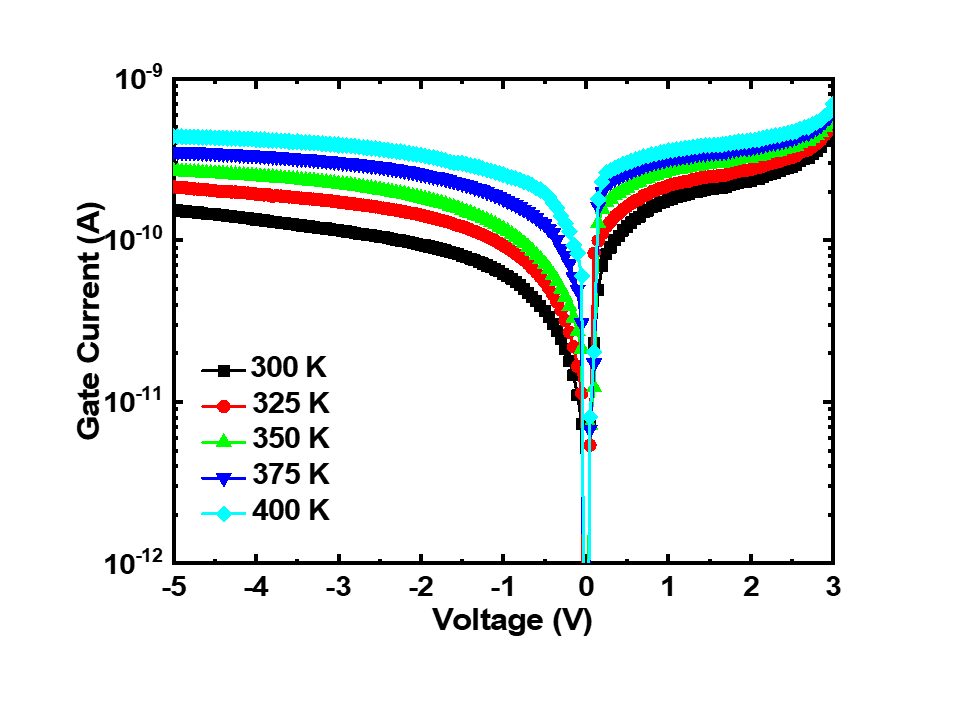Fig.3 shows the typical semi-logarithmic reverse and forward bias \textit{I}–\textit{V} characteristics of the Au/Ni/Al_{2}O_{3}/ AlGaN/GaN MOS diodes measured in the temperature range of 300 to 400 K at steps of 25 K by applying bias voltage from -5 to 3 V. The reverse gate leakage current at bias voltage of -1 V has been noted to be 6.60 {\times} 10^{-11}, 9.92 {\times} 10^{-11}, 1.25 {\times} 10^{-10}, 1.90 {\times} 10^{-10}, and 2.63 {\times} 10^{-10} A at temperatures of 300, 325, 350, 375 and 400 K, respectively. It is observed that the gate leakage current increases with increase in temperature. Then, in this study, the temperature dependence of current transport mechanisms at both reverse and forward bias voltage of Au/Ni/Al_{2}O_{3}/AlGaN/GaN MOS diode have been investigated. This is performed to determine the mechanisms dominating the current transport. The Poole-Frenkel emission, and Schottky emission has been analyzed in the context of the reverse bias voltage, and the power- law mechanism has been utilized for forward bias. Fig. 4. \textit{C}-\textit{V} characteristics of Au/Ni/Al_{2}O_{3}/AlGaN/GaN MOS diode structure in the frequency range of 1 KHz–1 MHz.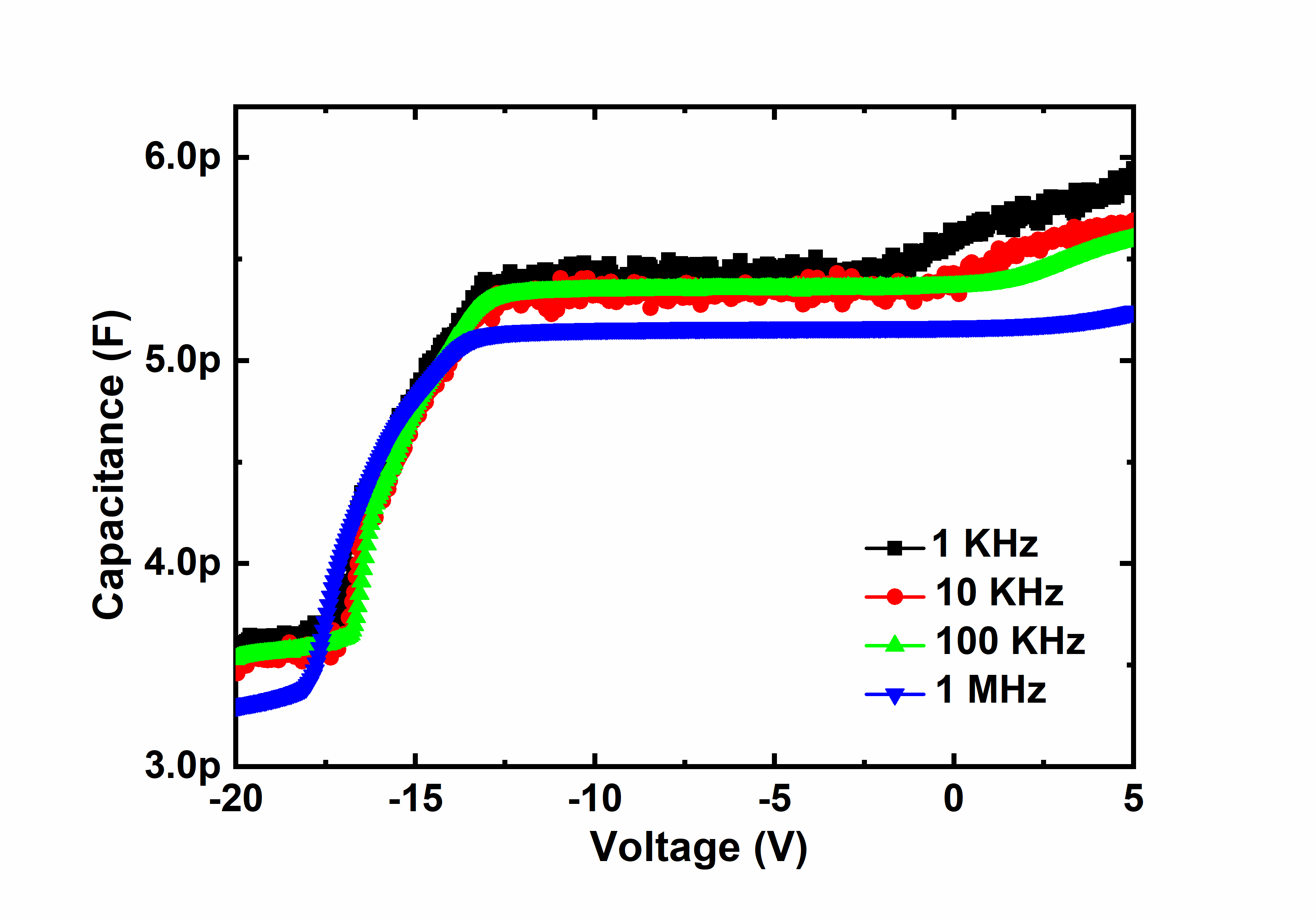Fig.4 shows the \textit{C}–\textit{V} characteristics of the Al_{2}O_{3}/ AlGaN/GaN diode structures with gate bias ranging from -20 to 5 V with a step of 0.05 V in the frequency range of 1 kHz to 1 MHz. The \textit{C}–\textit{V} curves obtained were peculiar having a two-step capacitance change, which is the characteristic feature of the MOS-HEMT structure having two interfaces [24,25]. The nearly flat capacitance \textit{C}$$_{\mathrm{2DEG}}$ indicates that the 2DEG accumulates at the AlGaN/GaN interface. On applying the positive bias, electrons start do distribute in the AlGaN layer, leading to an increase of capacitance to the insulator capacitance $\textit{C}$$_{\mathrm{a}}$$_{\mathrm{l2O3}}$. However, the capacitance of the gate oxide ($\textit{C}$$_{\mathrm{a}}$$_{\mathrm{l2O3}}$) corresponding to the 10-nm-thick Al$_{2}$O$_{3}$ was not observed till the measured bias of +5 V. The $\textit{C}$$_{\mathrm{2DEG}} is in accordance with the series capacitance of the 27-nm-thick AlGaN and the 25-nm-thick Al_{2}O_{3}. In negative bias range, as the gate bias nears the threshold voltage (\textit{V}$$_{\mathrm{th}}$), the depletion of 2DEG leads to sharp decrease of capacitance, corresponding to 2$^{\mathrm{nd }}$$\textit{C}–\textit{V} step. ##### (1) \begin{equation} n_{S}=\frac{1}{q\varepsilon A^{2}}\frac{C^{3}}{dC/dV} \end{equation} where \textit{A} denotes the Schottky diode area, \textit{q} is the electron charge, {\varepsilon} is the dielectric constant and d\textit{C}/d\textit{V} is the slope of the \textit{C}–\textit{V} characteristics. The inset in Fig.5 shows the depletion depth profile for the Au/Ni/Al_{2}O_{3}/ AlGaN/GaN MOS diode determined from the \textit{C}–\textit{V} characteristics (Fig.5) measured at 100 kHz. It can be seen that a plateau region of carrier concentration is prominent in the bulk GaN layer that marks enhanced background concentration at the interface of the two-step grown GaN. The 2DEG density (n_{\mathrm{s}}) at the AlGaN/GaN hetero-interface is found to be 7.15 {\times} 10^{14} cm^{2}. Fig. 5. \textit{C}–\textit{V} characteristics of Au/Ni/Al_{2}O_{3}/AlGaN/GaN MOS diode at 100 KHz with inset showing its corresponding depletion depth profile.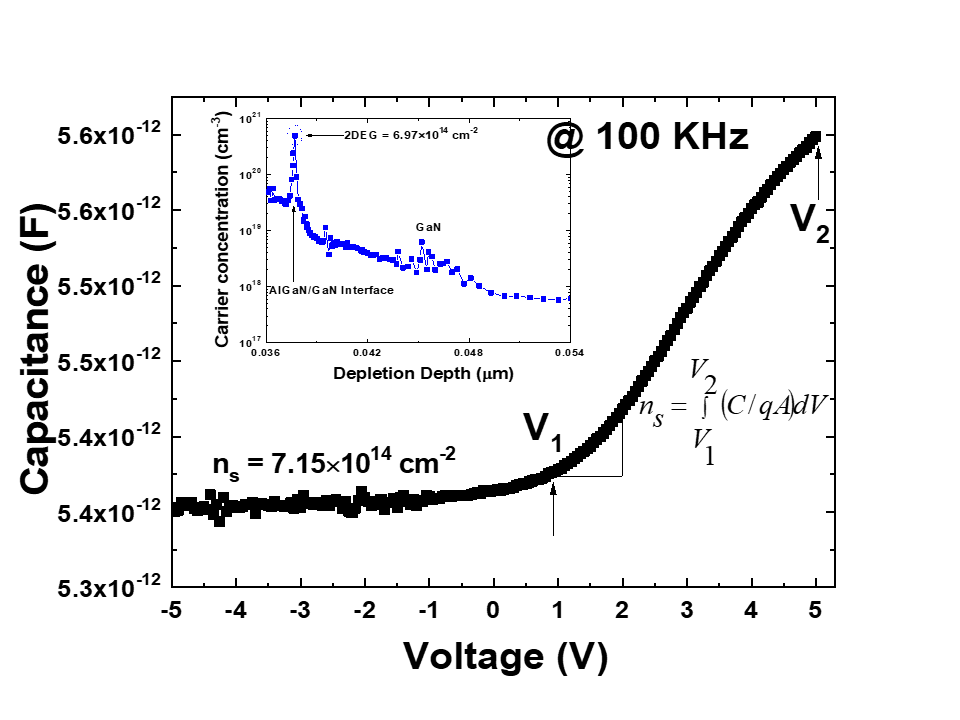The interface state density (\textit{N}$$_{ss}$) can be calculated from the capacitance-voltage measurements. At sufficiently high frequencies, the interface states are in equilibrium with the semiconductor and do not contribute to the capacitance as the charge at the interface states cannot follow the ac signal and any junction has only the space-charge capacitance ($\textit{C}$$_{sc}) in this case. At low frequencies, the interface state capacitance (\textit{C}$$_{it}$) adds up to the space-charge capacitance resulting in a higher total capacitance ($\textit{C}$). Hence, a subtraction of the space-charge capacitance obtained at high frequencies from the total capacitance measured at low frequencies yields the interface state capacitance ($\textit{C}$$_{it} = \textit{C}-\textit{C}$$_{SC}$). The interface state density ($\textit{N}$$_{SS}) can be obtained as : ##### (2) \begin{equation} N_{SS}=\frac{C_{it}}{qA}=\frac{1}{qA}\left(C- C_{SC}\right) \end{equation} where \textit{q} is the electron charge and \textit{A} is the area of the diode. The interface state density (\textit{N}$$_{SS}$) that is extracted from the measured high frequency (1 MHz) and low frequency (100 KHz) capacitance with applied bias is shown in Fig.6 for the Au/Ni/Al$_{2}$O$_{3}$/AlGaN/GaN MOS diode. It is observed that the value of $\textit{N}$$_{ss }is varied from 7.15 {\times}10^{14} to 1.12 {\times} 10^{15} eV^{-1} cm^{-2} as the applied reverse bias varies from 5 to 0 V. Fig. 6. Voltage dependence of interface state density (\textit{N}$$_{SS}$) distribution of Au/Ni/Al/Al$_{2}$O$_{3}$/AlGaN/GaN MOS diode at room temperature determined from $\textit{C}$–$\textit{V}$ characteristics.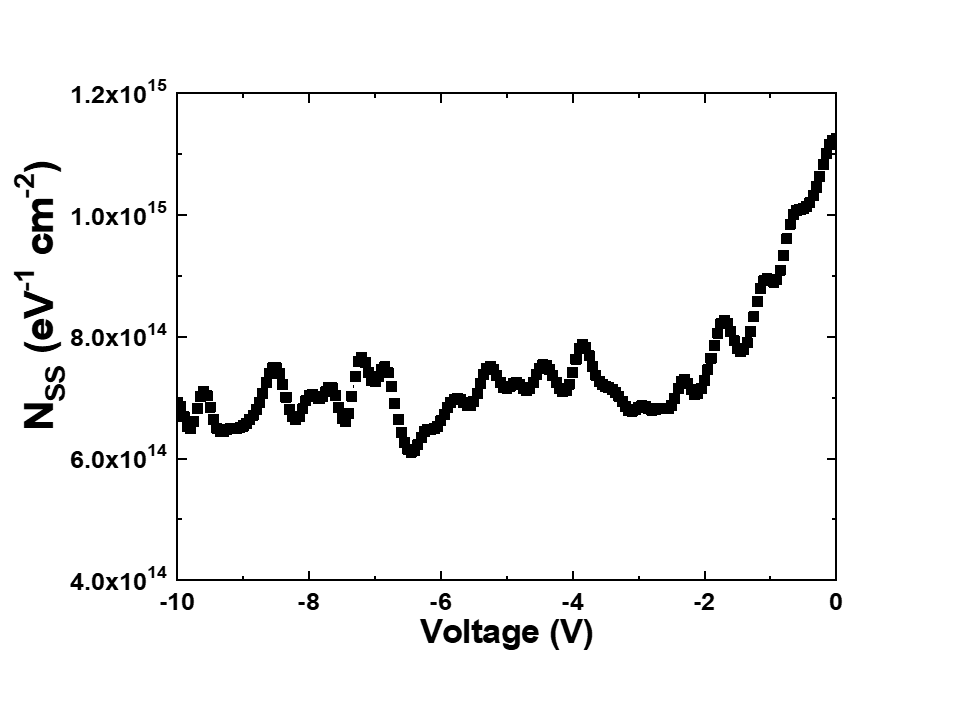## IV. CONCLUSION

The current transport mechanism dominating the reverse and forward gate leakage currents in Au/Ni/ Al$_{2}$O$_{3}$/AlGaN/GaN metal-oxide-semiconductor high electron mobility transistors (MOSHEMTs) have been investigated in the temperature range of 300–400 K. The reverse gate leakage current of the Au/Ni/Al$_{2}$O$_{3}$/AlGaN/ GaN MOS structure was dominated by Poole-Frenkel emission mechanism and Schottky emission mechanism in the lower and higher bias regions, irrespective of the measurement temperature. The forward log $\textit{I}$–log $\textit{V}$ characteristics of Au/Ni/Al$_{2}$O$_{3}$/AlGaN/GaN MOS structure indicated that the ohmic conduction dominated the carrier transport in the lower bias range. While the space-charge-limited current mechanism dominated the current conduction in the higher bias range in which the density of injected charge carriers is much larger than the thermally generated carrier. The results obtained in this study could be helpful in improving the gate leakage current of MOS-HEMT device.

### ACKNOWLEDGMENTS

This study was supported by the National Research Foundation of Korea (NRF) Grant (NRF-2017R1A2 B2003365) funded by the Ministry of Education, Republic of Korea, and by the transfer machine specialized lighting core technology development professional manpower training project (Project NO: N0001363) funded by the Ministry of Trade, Industry & Energy, Republic of Korea. This paper was also supported by the selection of research-oriented professor of Chonbuk National University in 2019.

### REFERENCES

1
Hori Y., Yatabe Z., Hashizume T., Dec 2013, Characterization of interface states in Al2O3/AlGaN/GaN structures for improved performance of high-electron-mobility transistors, J. Appl. Phys., Vol. 114, No. 24, pp. 244503-1-244503-82
Zhang H., Miller E. J., Yu E. T., Jan 2006, Analysis of leakage current mechanisms in Schottky contacts to GaN and Al0.25Ga0.75N/GaN grown by molecular-beam epitaxy, J. Appl. Phys., Vol. 99, No. 2, pp. 023703-1-023703-63
Selvaraj S. L., et al , Oct 2012, 1.4-kV Breakdown voltage for AlGaN/GaN high-electron-mobility transistors on silicon substrate, IEEE Electron Device Lett., Vol. 33, No. 10, pp. 1375-13774
Zhu J.-J., et al , Apr 2014, Investigation of gate leakage mechanism in Al2O3/Al0.55Ga0.45N/GaN metal-oxide-semiconductor high-electron-mobility transistors, Appl. Phys. Lett., Vol. 104, No. 15, pp. 153510-1-153510-45
Kordoš P., et al , Sep 2005, High-power SiO2/AlGaN/GaN metal-oxide-semiconductor heterostructure field-effect transistors, Appl. Phys. Lett., Vol. 87, No. 14, pp. 143501-1-143501-36
Liu Z. H., et al , Feb 2010, High microwave-noise performance of AlGaN/GAN MISHEMTs on silicon with Al2O3 Gate insulator grown by ALD, IEEE Electron Device Lett., Vol. 31, No. 2, pp. 96-987
Janardhanam V., et al , Jun 2017, Electrical properties of a Cu-germanide Schottky contact to n-type Ge depending on its microstructural evolution driven by rapid thermal annealing, Thin Solid Films, Vol. 632, No. 30, pp. 23-278
Schorder D. K., 2006, semiconductor material and Device Charaterization, third ed. John Wiley & Sons, Inc., New Jersey9
Samanta P., Mandal K. C., Jan 2017, Leakage current conduction, hole injection, and time-dependent dielectric breakdown of n-4H-SiC MOS capacitors during positive bias temperature stress, J. Appl. Phys., Vol. 121, No. 3, pp. 034501-1-034501-1310
Ahn K. H., Baik S., May 2002, Significant suppression of leakage current in (Ba,Sr)TiO3 thin films by Ni or Mn doping, J. Appl. Phys., Vol. 92, No. 5, pp. 2651-265411
Turuvekere S., et al , Oct 2013, Gate Leakage Mechanisms in AlGaN/GaN and AlInN/GaN HEMTs: comparison and modeling, IEEE Trans. Electron Devices, Vol. 60, No. 10, pp. 3157-316512
Xia L., et al , Mar 2013, On reverse gate leakage current of GaN high electron mobility transistors on silicon substrate, Appl. Phys. Lett., Vol. 102, No. 11, pp. 113510-1-113510-413
Karmalkar S., Satyan N., Sathaiya D. M., Feb 2006, On the resolution of the mechanism for reverse gate leakage in AlGaN/GaN HEMTs, IEEE Electron Device Lett, Vol. 27, No. 2, pp. 87-8914
Hasegawa H., Oyama S., Mar 2002, Mechanism of anomalous current transport in n-type GaN Schottky contacts, J. Vac. Sci. Technol. B, Vol. 20, No. 4, pp. 1647-165515
Yan D., etal , Oct 2010, On the reverse gate leakage current of AlGaN/GaN high electron mobility transistors, Appl. Phys. Lett., Vol. 97., No. 15, pp. 153503-1-153503-316
Ye P. D., et al , Jan 2005, GaN metal-oxide-semiconductor high-electron-mobility-transistor with atomic layer deposited Al2O3 as gate dielectric, Appl. Phys. Lett., Vol. 86, No. 6, pp. 063501-1-063501-317
Swain R., Jena K., Lenka T.R., Jun 2016, Modeling of forward gate leakage current in MOSHEMT using trap-assisted tunneling and Poole-Frenkel emission, IEEE. Trans. Electron Devices, Vol. 63, No. 6, pp. 2346-235218
Liu Z. H., et al , Apr 2011, Temperature-dependent forward gate current transport in atomic-layer-deposited Al2O3/AlGaN/GaN metal-insulator-semiconductor high electron mobility transistor, Appl. Phys. Lett., Vol. 98, No. 16, pp. 163501-1-163501-319
Reddy M. S. Pratap, Lee J. H., Jange J. S., Mar 2014, Electrical characteristics of TMAH-surface treated Ni/Au/Al2O3/GaN MIS Schottky structure, Electron. Mater. Lett., Vol. 10, No. 2, pp. 411-41620
Nishiguchi K., et al , Sep 2017, Current linearity and operation stability in Al2O3-gate AlGaN/GaN MOS high electron mobility transistors, Jpn. J. Appl. Phys., Vol. 56, No. 10, pp. 101001-1-101001-821
Hori Y., Yatabe Z., Hashizume T., Dec 2013, Characterization of interface states in Al2O3/AlGaN/GaN structure for improved performance of high-electron-mobility transistors, J. Appl. Phys., Vol. 114, No. 24, pp. 244503-1-244503-822
Osvald J., Stoklas R., Kordos P., May 2015, Extraction of interface traps density of Al2O3/AlGaN/GaN MIS heterostructure capacitance, Phys. Status Solidi B, Vol. 252, No. 5, pp. 996-100023
Shen L., et al , Jan 2016, Negative differential resistance in the I-V curves of Al2O3/AlGaN/GaN MIS structure, RSC Adv., Vol. 6, No. 7, pp. 5671-567624
Kim H., et al , Aug 2006, Passivation effects in Ni/AlGaN/GaN Schottky diodes by annealing, Appl. Phys. Lett., Vol. 89, No. 5, pp. 053516-1-053516-325
Lin J., et al , May 1990, Soft breakdown in titanium-silicided shallow source/drain junctions, IEEE Electron Device Lett., Vol. 11, No. 5, pp. 191-19326
Dinara S. M., et al , Apr 2015, Enhancement of two dimensional electron gas concentrations due to Si3N4 passivation on Al0.3Ga0.7N/GaN hetero-structure: strain and interface capacitance analysis, AIP Adv., Vol. 5, No. 4, pp. 047136-1-047136-1127
Mosbahi H., et al , Nov 2010, Electron traps studied in AlGaN/GaN HEMT on Si substrate using capacitance deep level transient spectroscopy, J. Optoelectronics Adv. Mater., Vol. 12, No. 11, pp. 2190-219328
Zhu Z.-F., et al , Aug 2017, Characterization of interface state density of Ni/p-GaN structures by capacitance/conductance-voltage-frequency measurements, Chin. Phys. Lett., Vol. 34, No. 9, pp. 097301-1-097301-529
Lee H. D., Apr 2000, Characterization of shallow silicided junctions for sub-quarter micron ULSI technology0–extraction of silicidation induced Schottky contact area, IEEE Trans. Electron Devices, Vol. 47, No. 4, pp. 762-76730
Lin J., et al , May 1990, Soft breakdown in titanium-silicided shallow source/drain junctions, IEEE Electron Device Lett., Vol. 11, No. 5, pp. 191-19331
Padovani F. A., Stratton R., Jul 1966, Field and thermionic-field emission in Schottky barriers, Solid State Electron, Vol. 9, No. 7, pp. 695-70732
Reddy V. Rajagopal, et al , May 2014, Electrical and current transport mechanisms of the Au/n-GaN Schottky structure with solution processed high-k BaTiO3 interlayer, J. Electron. Mater, Vol. 43, No. 9, pp. 3499-350733
Ocak Y. S., et al , Aug 2009, Current-voltage and capacitance-voltage characteristics of a Sn/Methylene Blue/p-Si Schottky diode, Synth. Met., Vol. 159, No. 15-16, pp. 1603-160734
Yildiz D. E., Apaydin D. H., Toppare L., Cirpan A., Jan 2017, Effect of layer thickness on the electrical parameters and conduction mechanisms of conjugated polymer-based heterojunction diode, J. Appl. Polym. Sci., Vol. 134, No. 19, pp. 44817-1-44817-735
Gupta S., Azam A., Akhtar J., Feb 2010, Experimental analysis of current conduction through thermally grown SiO2 on thick epitaxial 4H-SiC employing Poole-Frenkel mechanism, Pramana-J. Phys., Vol. 74, No. 2, pp. 325-33036
Janardhanam V., et al , Apr 2014, Electrical properties and carrier transport mechanism of Au/n-GaN Schottky contact modified using a Copper Pthalocyanine (CuPc) interlayer, Mater. Trans., Vol. 55, No. 5, pp. 758-76237
Lampert M. A., Mark P., 1970, Current injection in solids, Academic Press, New York, London38
Tyagi M., Tomar M., Jun 2015, Trap assisted space charge conduction in p-NiO/n-ZnO heterojunction diode, Mater. Res. Bull., Vol. 66, pp. 123-131## Author

Konepachith Ouduangvilai was born in Sayaboury, Laos, on November 09, 1993.

She received the B.S degree in physic from National University of Laos in 2015.

She is currently pursuing the M.S degree on semiconductor engineering at Chonbuk national University, South Korea.

Her research interests include the fabrication and characterization GaN MOS-HEMT devices.

Hoon-Ki Lee is a School of Semiconductor and Chemical Engineering, Semiconductor Physics Research Center (SPRC) Chonbuk University, Jeonju 561-756, Republic of Korea.

Vallivedu Janardhanam received the M.Sc., and Ph.D. in Physics, from Sri Venkateswara University, Tirupati, India, in 2003 and 2009, respectively.

Dr. V. Janardhanam joined as postdoctorate in the Semiconductor Physic Research Center, Chonbuk National University, in November 2009.

As author and co-author, he has published 79 articles in referred journals and has been the author and co-author of over 30 conference papers.

His current research interests include fabrication of Ohmic and Schottky contacts to germanium for post-silicon CMOS technologies, polymer-based contacts for Schottky diodes, and preparation of metal-polymer composite films for the development of organic-inorganic hybrid nanomaterials.

P. R. Sekhar Reddy was born in Andhra Pradesh, India, on 1990.

He received B.Sc. and M.Sc. degrees in Physics, from Sri Venkateswara University, Tirupati, India, in 2011 and 2014, respectively.

He is currently pursuing the Ph.D degree in Department of School of Semiconductor and Chemical Engineering, Semiconductor Physics Research Center, Chonbuk National University Republic of Korea.

His research interests include preparation of metal-polymer composite films for the development of organicinorganic hybrid nanomaterials.

Chel-Jong Choi was born in Korea, on June 16, 1974.

He received the B.S. degree in ceramic engineering from Hanyang University, Seoul, Korea, in 1997, and the M.S. and Ph.D. degrees in materials science engineering from the Gwangju Institute of Science and Technology (GIST), Gwangju Korea, in 1999 and 2003, respectively.

From 2003 to 2005, he was with the Samsung Advanced Institute of Technology (SAIT), Suwon, Korea, in the area of semiconductor-device characterization.

From 2005 to 2008, he worked with the Electronics and Telecommunications research Institute (ETRI), Daejon, Korea, where he was involved in the process integration of nano-scaled Schottky barrier MOSFETs.

Since 2008, he has been with Chonbuk National University, Jeonju, Korea.

His research interests include the novel nanoscaled Ge-and III-V-based electronic devices for the ultimate CMOS and post-CMOS technologies.

Kyu-Hwan Shim received his BS and MS degrees in materials science and engineering from Korea University in 1984 and 1986, respectively, and PhD degree from the University of Illinois at UrbanaChampaign (UIUC).

Meanwhile he joined the Electronics and Telecommunications Research Institute (ETRI) in 1986, where his major activities were focused on compound semiconductor processes and devices like GaAs MESFETs until 1992.

Thanks to ETRI’s program, he could study at UIUC for his PhD degree and specialize the epitaxial growth and device development of GaN-based Heterostructure.

For five years after 1999, while working as a principal research member, his efforts were devoted to SiGe HBTs, BiCMOS integrated circuits, and strained-silicon MOSFETs.

Then he moved to the Chonbuk National University (CBNU) to become a professor in 2004 and continued his researched on SiGe and related semiconductor materials, processes, and devices in his lab Intelligent Semiconductor Research Lab (ISRL).

At the same time, he started to serve as a CEO for a new lab-based venture company, Sigetronics Ltd. Established inside the CBNU campus, where various semiconductor devices such as Zener diode (for LEDs), TVS, ULC-TVS, ESD/EMI Filter, SBD, FRD, Power-FETs have been developed and commercialized.

His research is focused mostly on the epitaxial growth of germanium on silicon substrates (Ge-on-Si) and its application for future electrical and the emitters/receivers of optical signals for communications and image signal processing as well.

He wants to assist for a new generation started on the basis of GaN, Ge, Si in every academic and industrial sectors.